Courses

# Design of Control Systems - MCQ Test

## 20 Questions MCQ Test GATE ECE (Electronics) 2022 Mock Test Series | Design of Control Systems - MCQ Test

Description
This mock test of Design of Control Systems - MCQ Test for Electronics and Communication Engineering (ECE) helps you for every Electronics and Communication Engineering (ECE) entrance exam. This contains 20 Multiple Choice Questions for Electronics and Communication Engineering (ECE) Design of Control Systems - MCQ Test (mcq) to study with solutions a complete question bank. The solved questions answers in this Design of Control Systems - MCQ Test quiz give you a good mix of easy questions and tough questions. Electronics and Communication Engineering (ECE) students definitely take this Design of Control Systems - MCQ Test exercise for a better result in the exam. You can find other Design of Control Systems - MCQ Test extra questions, long questions & short questions for Electronics and Communication Engineering (ECE) on EduRev as well by searching above.
QUESTION: 1

Solution:
QUESTION: 2

Solution:
QUESTION: 3

### The transfer function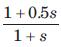represent a

Solution:
QUESTION: 4

A lag compensation network
(a) increases the gain of the original network without affecting stability.
(b) reduces the steady state error.
(c) reduces the speed of response
(d) permits the increase of gain of phase margin is acceptable.
In the above statements, which are correct

Solution:
QUESTION: 5

Phase lag controller:

Solution:

Phase lag controller is the integral controller that creates the phase lag and does not affect the value of the damping factor and that tries to reduce the steady state error.

QUESTION: 6

Consider the List I and List II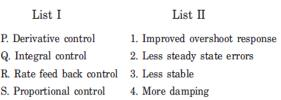The correct match is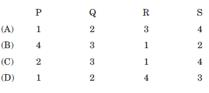Solution:
QUESTION: 7

A control system in which the control action is somehow dependent on the output is known as

Solution:
QUESTION: 8

The transfer function of a compensating network is of form (1 + αTs)/(1 + Ts). If this is a phase–Lag network, the value of α  should be

Solution:
QUESTION: 9

The poll–zero configuration of a phase–lead compensator is given by

Solution:
QUESTION: 10

While designing controller, the advantage of pole–zero cancellation is

Solution:
QUESTION: 11

A proportional controller leads to

Solution:
QUESTION: 12

The transfer function of a phase compensator is given by (1 + αTs) /(1 + Ts) where α > 1 and T >  0. The maximum phase shift provided by a such compensator is

Solution:
QUESTION: 13

For an electrically heated temperature controlled liquid heater, the best controller is

Solution:
QUESTION: 14

When phase-lag compensation is used in a system, gain crossover frequency, band width and undamped frequency are respectively :

Solution:
QUESTION: 15

A process with open–loop model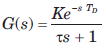is controlled by a PID controller. For this purpose
(A) the derivative mode improves transient performance
(B) the derivative mode improves steady state performance
(C) the integral mode improves transient performance
(D) the integral mode improves steady state performance.
The correct statements are

Solution:
QUESTION: 16

A lead compensating network
(a) improves response time
(b) stabilizes the system with low phase margin
(c) enables moderate increase in gain without affecting stability.
(d) increases resonant frequency
In the above statements, correct are

Solution:
QUESTION: 17

A Lag network for compensation normally consists of

Solution:
QUESTION: 18

The pole–zero plot given in fig. is that of a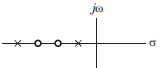Solution:
QUESTION: 19

The correct sequence of steps needed to improve system stability is

Solution:
QUESTION: 20

In a derivative error compensation

Solution: